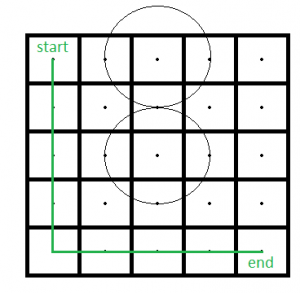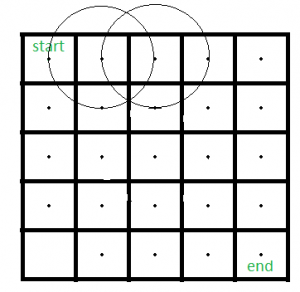# Path in a Rectangle with Circles

There is a m*n rectangular matrix whose top-left(start) location is (1, 1) and bottom-right(end) location is (m*n). There are k circles each with radius r. Find if there is any path from start to end without touching any circle.

The input contains values of m, n, k, r and two array of integers X and Y, each of length k. (X[i], Y[i]) is the centre of ith circle.

Source : Directi Interview

Examples:

```Input1 : m = 5, n = 5, k = 2, r = 1,
X = {1, 3}, Y = {3, 3}
Output1 : PossibleHere is a path from start to end point.

Input2 : m = 5, n = 5, k = 2, r = 1,
X = {1, 1}, Y = {2, 3}.
Output2 : Not Possible```

## Recommended: Please try your approach on {IDE} first, before moving on to the solution.

Approach : Check if the centre of a cell (i, j) of the rectangle comes within any of the circles then do not traverse through that cell and mark that as ‘blocked’. Mark rest of the cells initially as ‘unvisited’. Then use BFS to find out shortest path of each cell from starting position. If the end cell is visited then we will return “Possible” otherwise “Not Possible”.

Algorithm :

1. Take an array of size m*n. Initialize all the cells to 0.
2. For each cell of the rectangle check whether it comes within any circle or not (by calculating the distance of that cell from each circle). If it comes within any circle then change the value of that cell to -1(‘blocked’).
3. Now, apply BFS from the starting cell and if a cell can be reached then change the value of that cell to 1.
4. If the value of the ending cell is 1, then return ‘Possible’, otherwise return ‘Not Possible’.

## C++

 `// C++ program to find out path in ` `// a rectangle containing circles. ` `#include ` `#include ` `#include ` ` `  `using` `namespace` `std; ` ` `  `// Function to find out if there is ` `// any possible path or not. ` `bool` `isPossible(``int` `m, ``int` `n, ``int` `k, ``int` `r,  ` `                ``vector<``int``> X, vector<``int``> Y)  ` `{ ` `  ``// Take an array of m*n size and ` `  ``// initialize each element to 0. ` `  ``int` `rect[m][n] = {0}; ` ` `  `  ``// Now using Pythagorean theorem find if a ` `  ``// cell touches or within any circle or not. ` `  ``for` `(``int` `i = 0; i < m; i++) { ` `    ``for` `(``int` `j = 0; j < n; j++) { ` `      ``for` `(``int` `p = 0; p < k; p++) { ` `        ``if` `(``sqrt``((``pow``((X[p] - 1 - i), 2) +  ` `            ``pow``((Y[p] - 1 - j), 2))) <= r)  ` `        ``{ ` `          ``rect[i][j] = -1; ` `        ``} ` `      ``} ` `    ``} ` `  ``} ` `   `  `  ``// If the starting cell comes within ` `  ``// any circle return false. ` `  ``if` `(rect == -1) ` `    ``return` `false``; ` ` `  `  ``// Now use BFS to find if there ` `  ``// is any possible path or not. ` ` `  `  ``// Initialize the queue which holds ` `  ``// the discovered cells whose neighbors ` `  ``// are not discovered yet. ` `  ``vector> qu; ` ` `  `  ``rect = 1; ` `  ``qu.push_back({0, 0}); ` `   `  `  ``// Discover cells until queue is not empty ` `  ``while` `(!qu.empty()) { ` `    ``vector<``int``> arr = qu.front(); ` `    ``qu.erase(qu.begin()); ` `    ``int` `elex = arr; ` `    ``int` `eley = arr; ` ` `  `    ``// Discover the eight adjacent nodes. ` `    ``// check top-left cell ` `    ``if` `((elex > 0) && (eley > 0) &&  ` `        ``(rect[elex - 1][eley - 1] == 0))  ` `    ``{ ` `      ``rect[elex - 1][eley - 1] = 1; ` `      ``vector<``int``> v = {elex - 1, eley - 1}; ` `      ``qu.push_back(v); ` `    ``} ` `     `  `    ``// check top cell ` `    ``if` `((elex > 0) &&  ` `        ``(rect[elex - 1][eley] == 0)) ` `    ``{ ` `      ``rect[elex - 1][eley] = 1; ` `      ``vector<``int``> v = {elex - 1, eley}; ` `      ``qu.push_back(v); ` `    ``} ` `     `  `    ``// check top-right cell ` `    ``if` `((elex > 0) && (eley < n - 1) &&  ` `        ``(rect[elex - 1][eley + 1] == 0))  ` `    ``{ ` `      ``rect[elex - 1][eley + 1] = 1; ` `      ``vector<``int``> v = {elex - 1, eley + 1}; ` `      ``qu.push_back(v); ` `    ``} ` `     `  `    ``// check left cell ` `    ``if` `((eley > 0) &&  ` `        ``(rect[elex][eley - 1] == 0))  ` `    ``{ ` `      ``rect[elex][eley - 1] = 1; ` `      ``vector<``int``> v = {elex, eley - 1}; ` `      ``qu.push_back(v); ` `    ``} ` `     `  `    ``// check right cell ` `    ``if` `((eley > n - 1) &&  ` `        ``(rect[elex][eley + 1] == 0)) ` `    ``{ ` `      ``rect[elex][eley + 1] = 1; ` `      ``vector<``int``> v = {elex, eley + 1}; ` `      ``qu.push_back(v); ` `    ``} ` `     `  `    ``// check bottom-left cell ` `    ``if` `((elex < m - 1) && (eley > 0) &&  ` `        ``(rect[elex + 1][eley - 1] == 0)) ` `    ``{ ` `      ``rect[elex + 1][eley - 1] = 1; ` `      ``vector<``int``> v = {elex + 1, eley - 1}; ` `      ``qu.push_back(v); ` `    ``} ` `     `  `    ``// check bottom cell ` `    ``if` `((elex < m - 1) &&  ` `        ``(rect[elex + 1][eley] == 0)) ` `    ``{ ` `      ``rect[elex + 1][eley] = 1; ` `      ``vector<``int``> v = {elex + 1, eley}; ` `      ``qu.push_back(v); ` `    ``} ` `     `  `    ``// check bottom-right cell ` `    ``if` `((elex < m - 1) && (eley < n - 1) &&  ` `        ``(rect[elex + 1][eley + 1] == 0))  ` `    ``{ ` `      ``rect[elex + 1][eley + 1] = 1; ` `      ``vector<``int``> v = {elex + 1, eley + 1}; ` `      ``qu.push_back(v); ` `    ``} ` `  ``} ` ` `  `  ``// Now if the end cell (i.e. bottom right cell) ` `  ``// is 1(reachable) then we will send true. ` `  ``return` `(rect[m - 1][n - 1] == 1); ` `} ` ` `  `// Driver Program ` `int` `main() { ` `     `  `  ``// Test case 1 ` `  ``int` `m1 = 5, n1 = 5, k1 = 2, r1 = 1; ` `  ``vector<``int``> X1 = {1, 3}; ` `  ``vector<``int``> Y1 = {3, 3}; ` `  ``if` `(isPossible(m1, n1, k1, r1, X1, Y1)) ` `    ``cout << ``"Possible"` `<< endl; ` `  ``else` `    ``cout << ``"Not Possible"` `<< endl; ` ` `  `  ``// Test case 2 ` `  ``int` `m2 = 5, n2 = 5, k2 = 2, r2 = 1; ` `  ``vector<``int``> X2 = {1, 1}; ` `  ``vector<``int``> Y2 = {2, 3}; ` `  ``if` `(isPossible(m2, n2, k2, r2, X2, Y2)) ` `    ``cout << ``"Possible"` `<< endl; ` `  ``else` `    ``cout << ``"Not Possible"` `<< endl; ` `     `  `  ``return` `0; ` `} `

## Python3

 `# Python3 program to find out path in  ` `# a rectangle containing circles. ` `import` `math  ` `import` `queue ` ` `  `# Function to find out if there is  ` `# any possible path or not.  ` `def` `isPossible(m, n, k, r, X, Y): ` `     `  `    ``# Take an array of m*n size and  ` `    ``# initialize each element to 0.  ` `    ``rect ``=` `[[``0``] ``*` `n ``for` `i ``in` `range``(m)] ` ` `  `    ``# Now using Pythagorean theorem find if a  ` `    ``# cell touches or within any circle or not. ` `    ``for` `i ``in` `range``(m): ` `        ``for` `j ``in` `range``(n): ` `            ``for` `p ``in` `range``(k): ` `                ``if` `(math.sqrt((``pow``((X[p] ``-` `1` `-` `i), ``2``) ``+`  `                               ``pow``((Y[p] ``-` `1` `-` `j), ``2``))) <``=` `r): ` `                    ``rect[i][j] ``=` `-``1` `     `  `    ``# If the starting cell comes within  ` `    ``# any circle return false.  ` `    ``if` `(rect[``0``][``0``] ``=``=` `-``1``):  ` `        ``return` `False` ` `  `    ``# Now use BFS to find if there  ` `    ``# is any possible path or not.  ` ` `  `    ``# Initialize the queue which holds  ` `    ``# the discovered cells whose neighbors  ` `    ``# are not discovered yet.  ` `    ``qu ``=` `queue.Queue()  ` ` `  `    ``rect[``0``][``0``] ``=` `1` `    ``qu.put([``0``, ``0``])  ` `     `  `    ``# Discover cells until queue is not empty  ` `    ``while` `(``not` `qu.empty()): ` `        ``arr ``=` `qu.get() ` `        ``elex ``=` `arr[``0``]  ` `        ``eley ``=` `arr[``1``]  ` ` `  `        ``# Discover the eight adjacent nodes.  ` `        ``# check top-left cell  ` `        ``if` `((elex > ``0``) ``and` `(eley > ``0``) ``and`  `            ``(rect[elex ``-` `1``][eley ``-` `1``] ``=``=` `0``)): ` `            ``rect[elex ``-` `1``][eley ``-` `1``] ``=` `1` `            ``v ``=` `[elex ``-` `1``, eley ``-` `1``]  ` `            ``qu.put(v) ` `     `  `        ``# check top cell  ` `        ``if` `((elex > ``0``) ``and`  `            ``(rect[elex ``-` `1``][eley] ``=``=` `0``)): ` `            ``rect[elex ``-` `1``][eley] ``=` `1` `            ``v ``=` `[elex ``-` `1``, eley]  ` `            ``qu.put(v) ` `     `  `        ``# check top-right cell  ` `        ``if` `((elex > ``0``) ``and` `(eley < n ``-` `1``) ``and`  `            ``(rect[elex ``-` `1``][eley ``+` `1``] ``=``=` `0``)): ` `            ``rect[elex ``-` `1``][eley ``+` `1``] ``=` `1` `            ``v ``=` `[elex ``-` `1``, eley ``+` `1``]  ` `            ``qu.put(v) ` `     `  `        ``# check left cell  ` `        ``if` `((eley > ``0``) ``and`  `            ``(rect[elex][eley ``-` `1``] ``=``=` `0``)): ` `            ``rect[elex][eley ``-` `1``] ``=` `1` `            ``v ``=` `[elex, eley ``-` `1``]  ` `            ``qu.put(v) ` `     `  `        ``# check right cell  ` `        ``if` `((eley > n ``-` `1``) ``and`  `            ``(rect[elex][eley ``+` `1``] ``=``=` `0``)): ` `            ``rect[elex][eley ``+` `1``] ``=` `1` `            ``v ``=` `[elex, eley ``+` `1``]  ` `            ``qu.put(v) ` `     `  `        ``# check bottom-left cell  ` `        ``if` `((elex < m ``-` `1``) ``and` `(eley > ``0``) ``and`  `            ``(rect[elex ``+` `1``][eley ``-` `1``] ``=``=` `0``)): ` `            ``rect[elex ``+` `1``][eley ``-` `1``] ``=` `1` `            ``v ``=` `[elex ``+` `1``, eley ``-` `1``]  ` `            ``qu.put(v) ` `     `  `        ``# check bottom cell  ` `        ``if` `((elex < m ``-` `1``) ``and`  `            ``(rect[elex ``+` `1``][eley] ``=``=` `0``)): ` `            ``rect[elex ``+` `1``][eley] ``=` `1` `            ``v ``=` `[elex ``+` `1``, eley] ` `            ``qu.put(v) ` `     `  `        ``# check bottom-right cell  ` `        ``if` `((elex < m ``-` `1``) ``and` `(eley < n ``-` `1``) ``and`  `            ``(rect[elex ``+` `1``][eley ``+` `1``] ``=``=` `0``)): ` `            ``rect[elex ``+` `1``][eley ``+` `1``] ``=` `1` `            ``v ``=` `[elex ``+` `1``, eley ``+` `1``]  ` `            ``qu.put(v)  ` ` `  `    ``# Now if the end cell (i.e. bottom right cell)  ` `    ``# is 1(reachable) then we will send true.  ` `    ``return` `(rect[m ``-` `1``][n ``-` `1``] ``=``=` `1``) ` ` `  `# Driver Code ` `if` `__name__ ``=``=` `'__main__'``: ` ` `  `    ``# Test case 1  ` `    ``m1 ``=` `5` `    ``n1 ``=` `5` `    ``k1 ``=` `2` `    ``r1 ``=` `1` `    ``X1 ``=` `[``1``, ``3``] ` `    ``Y1 ``=` `[``3``, ``3``] ` `    ``if` `(isPossible(m1, n1, k1, r1, X1, Y1)):  ` `        ``print``(``"Possible"``)  ` `    ``else``: ` `        ``print``(``"Not Possible"``)  ` ` `  `    ``# Test case 2  ` `    ``m2 ``=` `5` `    ``n2 ``=` `5` `    ``k2 ``=` `2` `    ``r2 ``=` `1` `    ``X2 ``=` `[``1``, ``1``] ` `    ``Y2 ``=` `[``2``, ``3``] ` `    ``if` `(isPossible(m2, n2, k2, r2, X2, Y2)):  ` `        ``print``(``"Possible"``)  ` `    ``else``: ` `        ``print``(``"Not Possible"``) ` ` `  `# This code is contributed by PranchalK `

Output:

```Possible
Not Possible
```

Time Complexity : It takes O(m*n*k) time to compute whether a cell is within or not in any circle. And it takes O(V+E) time in BFS. Here, number of edges in m*n grid is m*(n-1)+n*(m-1) and vertices m*n. So it takes O(m*n) time in DFS. Hence, the time complexity is O(m*n*k). The complexity can be improved if we iterate through each circles and mark -1 the cells which are coming within it.

Don’t stop now and take your learning to the next level. Learn all the important concepts of Data Structures and Algorithms with the help of the most trusted course: DSA Self Paced. Become industry ready at a student-friendly price.

My Personal Notes arrow_drop_upCheck out this Author's contributed articles.

If you like GeeksforGeeks and would like to contribute, you can also write an article using contribute.geeksforgeeks.org or mail your article to contribute@geeksforgeeks.org. See your article appearing on the GeeksforGeeks main page and help other Geeks.

Please Improve this article if you find anything incorrect by clicking on the "Improve Article" button below.

Improved By : PranchalKatiyar